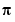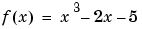MATLAB Function Referencefminbnd

Minimize a function of one variable on a fixed interval

Syntax

• ```x = fminbnd(fun,x1,x2)
x = fminbnd(fun,x1,x2,options)
[x,fval] = fminbnd(...)
[x,fval,exitflag] = fminbnd(...)
[x,fval,exitflag,output] = fminbnd(...)
```

Description

`fminbnd` finds the minimum of a function of one variable within a fixed interval.

```x = fminbnd(fun,x1,x2) ``` returns a value `x` that is a local minimizer of the function that is described in `fun` in the interval `x1 <= x <= x2`. `fun` is a function handle. See Function Handles in the MATLAB Programming documentation for more information.

Parameterizing Functions Called by Function Functions, in the MATLAB mathematics documentation, explains how to provide additional parameters to the function `fun`, if necessary.

```x = fminbnd(fun,x1,x2,options) ``` minimizes with the optimization parameters specified in the structure `options`. You can define these parameters using the `optimset` function. `fminbnd` uses these `options` structure fields:

 `Display` Level of display. `'off'` displays no output; `'iter'` displays output at each iteration; `'final'` displays just the final output; `'notify'` (default) displays output only if the function does not converge. `FunValCheck` Check whether objective function values are valid. `'on'` displays a warning when the objective function returns a value that is `complex` or `NaN`. `'off'` displays no warning. `MaxFunEvals` Maximum number of function evaluations allowed. `MaxIter` Maximum number of iterations allowed. `OutputFcn` Specify a user-defined function that the optimization function calls at each iteration. `TolX` Termination tolerance on `x`.

```[x,fval] = fminbnd(...) ``` returns the value of the objective function computed in `fun` at `x`.

```[x,fval,exitflag] = fminbnd(...) ``` returns a value `exitflag` that describes the exit condition of `fminbnd`:

 `1` `fminbnd` converged to a solution `x` based on `options.TolX.` `0` Maximum number of function evaluations or iterations was reached. `-1` Algorithm was terminated by the output function. `-2` Bounds are inconsistent (`ax > bx`).

```[x,fval,exitflag,output] = fminbnd(...) ``` returns a structure `output` that contains information about the optimization:

 `output.algorithm` Algorithm used `output.funcCount` Number of function evaluations `output.iterations` Number of iterations `output.message` Exit message

Arguments

`fun` is the function to be minimized. `fun` accepts a scalar `x` and returns a scalar `f`, the objective function evaluated at `x`. The function `fun` can be specified as a function handle for an M-file function

• ```x = fminbnd(@myfun,x1,x2);
```

where `myfun.m` is an M-file function such as

• ```function f = myfun(x)
f = ...         % Compute function value at x.
```

or as a function handle for an anonymous function:

• ```x = fminbnd(@(x) sin(x*x),x1,x2);
```

Other arguments are described in the syntax descriptions above.

Examples

`x = fminbnd(@cos,3,4)` computesto a few decimal places and gives a message on termination.

• ```[x,fval,exitflag] = ...
fminbnd(@cos,3,4,optimset('TolX',1e-12,'Display','off'))
```

computesto about 12 decimal places, suppresses output, returns the function value at `x`, and returns an `exitflag` of 1.

The argument `fun` can also be a function handle for an anonymous function. For example, to find the minimum of the functionon the interval `(0,2)`, create an anonymous function `f`

• ```f = @(x)x.^3-2*x-5;
```

Then invoke `fminbnd` with

• ```x = fminbnd(f`,` 0`,` 2)
```

The result is

• ```x =
0.8165
```

The value of the function at the minimum is

• ```y = f(x)

y =
-6.0887
```

If `fun` is parameterized, you can use anonymous functions to capture the problem-dependent parameters. For example, suppose you want to minimize the objective function `myfun` defined by the following M-file function.

• ```function f = myfun(x,a)
f = (x - a)^2;
```

Note that `myfun` has an extra parameter `a`, so you cannot pass it directly to `fminbind`. To optimize for a specific value of `a`, such as `a = 1.5`.

1. Assign the value to `a`.
• ```a = 1.5; % define parameter first
```
2. Call `fminbnd` with a one-argument anonymous function that captures that value of `a` and calls `myfun` with two arguments:
• ```x = fminbnd(@(x) myfun(x,a),0,1)
```

Algorithm

`fminbnd` is an M-file. The algorithm is based on golden section search and parabolic interpolation. Unless the left endpoint x1 is very close to the right endpoint x2, `fminbnd` never evaluates `fun` at the endpoints, so `fun` need only be defined for x in the interval x1 < x < x2. If the minimum actually occurs at x1 or x2, `fminbnd` returns an interior point at a distance of no more than `2*TolX` from x1 or x2, where `TolX` is the termination tolerance. See  or  for details about the algorithm.

Limitations

The function to be minimized must be continuous. `fminbnd` may only give local solutions.

`fminbnd` often exhibits slow convergence when the solution is on a boundary of the interval.

`fminbnd` only handles real variables.

See Also

`fminsearch`, `fzero`, `optimset`, `function_handle` (`@`), anonymous functions

References

  Forsythe, G. E., M. A. Malcolm, and C. B. Moler, Computer Methods for Mathematical Computations, Prentice-Hall, 1976.

  Brent, Richard. P., Algorithms for Minimization without Derivatives, Prentice-Hall, Englewood Cliffs, New Jersey, 1973

© 1994-2005 The MathWorks, Inc.